# How to AutoSum Multiple Rows and Columns in Excel

This post will guide you how to AutoSum function to sum multiple rows and columns quickly in Excel.

## AutoSum Multiple Rows and Columns

Assuming that you have a list of data in range:A1:C5, in which contain numeric values. And you want to sum those numbers at once. How to do it. You can use the AutoSum feature to calculate the sum values of those numbers in range A1:C5 quickly. Just do the following steps:

#1 select the range of cells in which you want to calculate the sum values.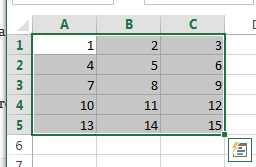#2 go to FORMULAS tab, click AutoSum command under Function Library group.  Or you can go to HOME tab, click AutoSUM button under Editing group.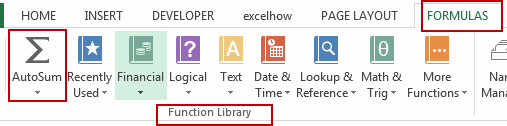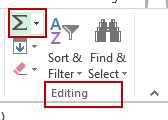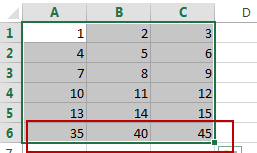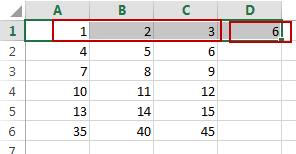You would notice that the sum values are calculated and appear in the last row and in the last column of the selected range.

## Comments

So empty here ... leave a comment!
Sidebar